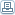# Fibonacci Numbers and Forex Trading - Is The Theory Legitimate?

 By: Ian ArmstrongYou might not have heard of Leonardo Fibonacci. He was a well known mathematician who lived in Italy between 1175 and 1250. He's responsible for a number of significant contributions to mathematics as we know it, including the introduction of the decimal system to Europe. He also studied a particular sequence of numbers, now known as the Fibonacci Sequence. This sequence starts with zero and one. Each new number is the sum of the two numbers that have gone before. That means that the second number is one. Since one and zero added together equal one, this is the third number. The first numbers in the Fibonacci sequence are zero, one, one, two, three, five, eight, thirteen, twenty-one, thirty-four, fifty-five, eight-nine, a hundred and forty-four, etc. The sequence theoretically continues into infinity. The interesting thing about the numbers in this sequence is how frequently they turn up in the world around us.They even show up in Forex trading. Ratios found in the Fibonacci sequence can be seen in currency price moments. They also appear in the price movements of stocks and other types of investment. The big three numbers you should pay attention to in Forex trading are 0.382, 0.5, and 0.618. There are plenty of other numbers, but these are the most important. That's because they're used to calculate what are called retracement levels. These are used by a lot of traders to decide when they should place their buy and sell orders. This works as follows:If you assume that the price of a pair of currencies, or even of a company's stock is trending upward, you'll also need to assum that prices will hit a peak. They'll then go into a temporary reversal, and resume the trend after a little while. This reversal is where Fibonacci numbers come in handy. That's because the price of currencies that are trending can be expected to reverse back into a Fibonacci number, then bounce back, contining the trend. A correct forecast can allow you to buy in just before the upward trend starts up again. You could make a bit profit this way.Use an online trading platform that can chart these numbers for you. Draw a line from a particular low point to a high point, and the retracement levels should be mapped automatically on a chart. Of course, it's not as simple as just trading when the price is the same as a Fibonacci number. There are plenty of other things to remember. For one thing, you don't know what level the price will stop at. Choosing .382 when it could drop to .618 will cause you to lose a lot. Also, it's important to choose the right low and high points to make sure the retracement levels are correct. Also, even though they can be incredibly accurate at times, Fibonacci numbers don't always work a hundred percent of the time. There are two many variables at work in the Forex market. You can't rely on just one method to find out what the prices are going to do.